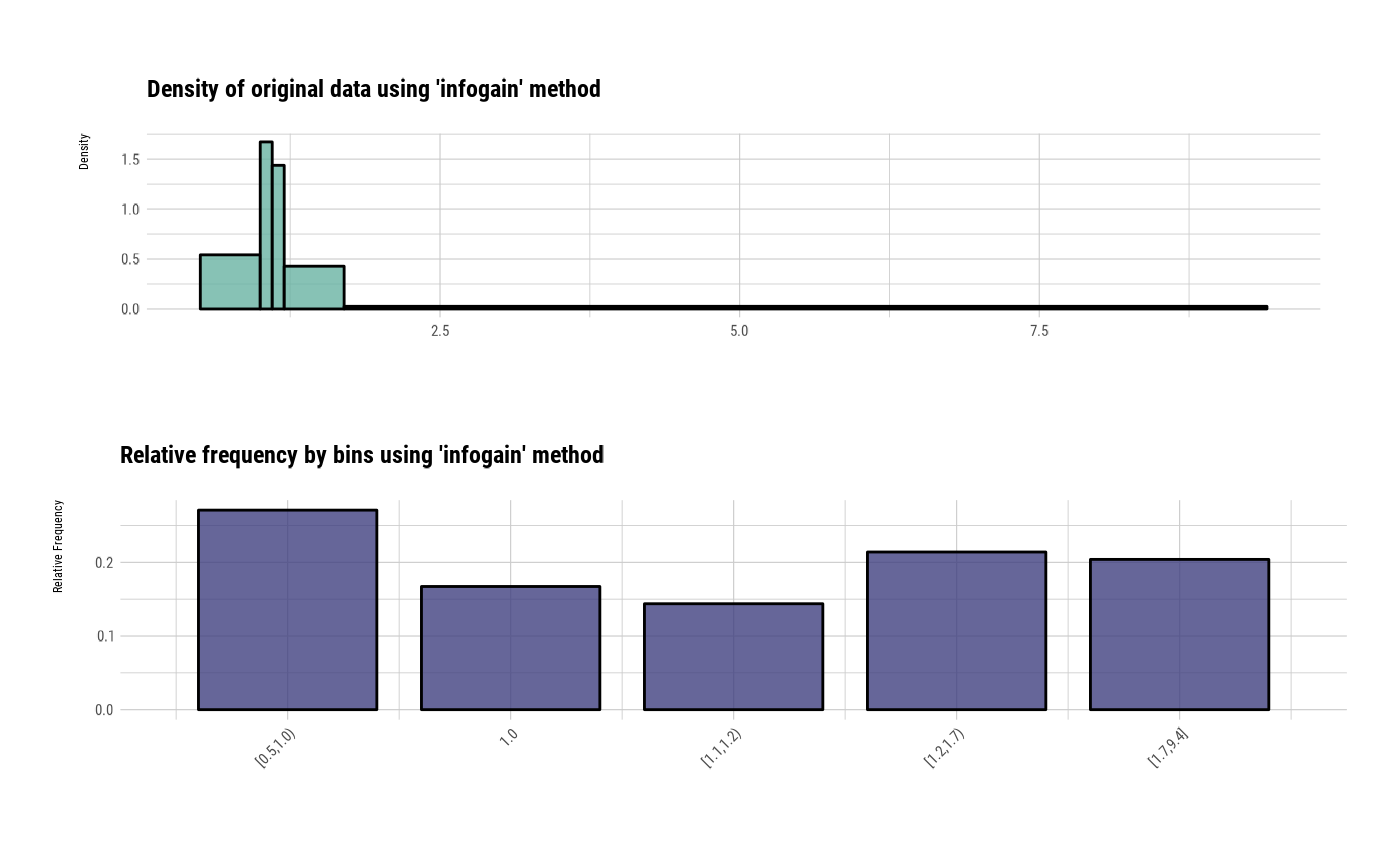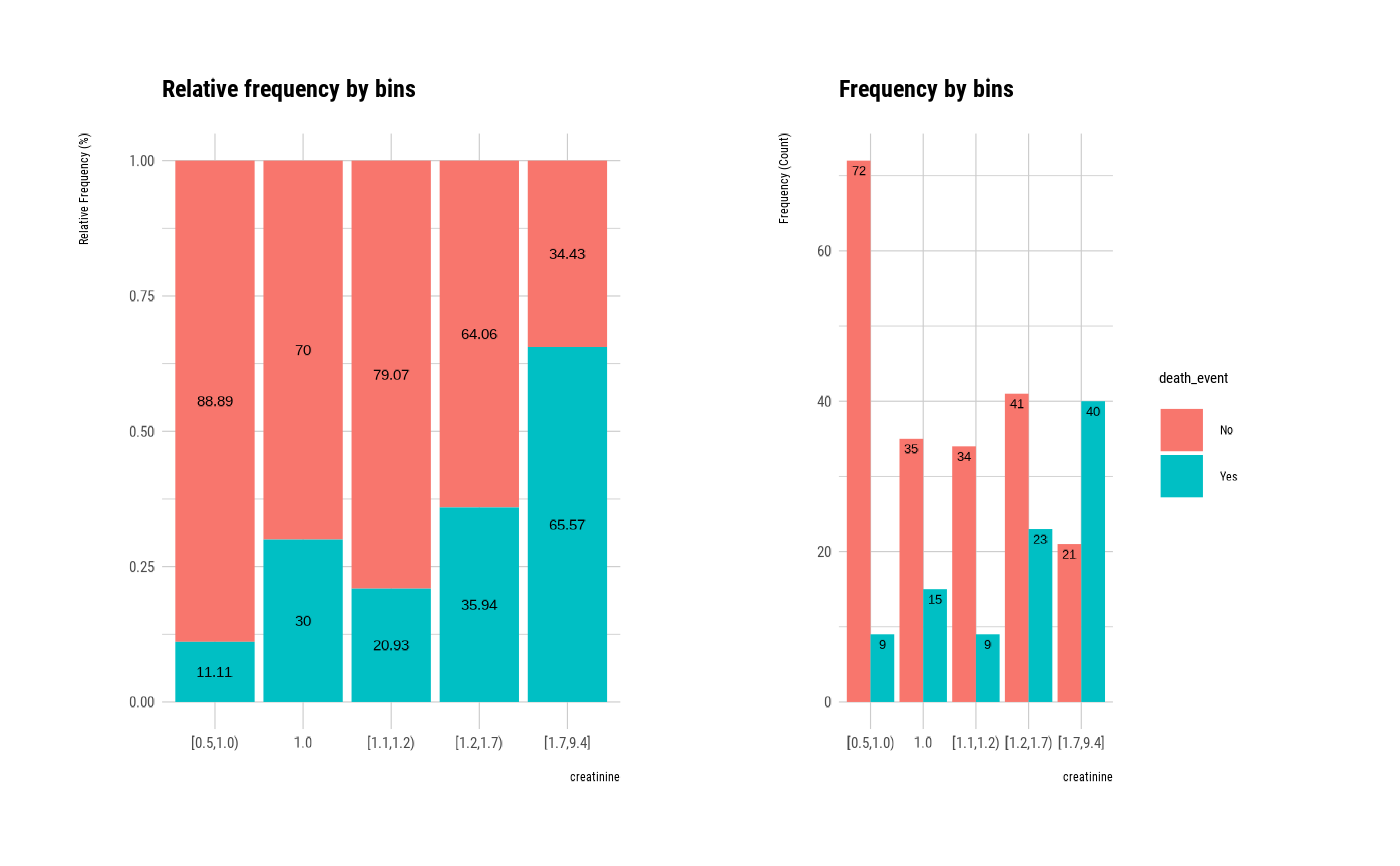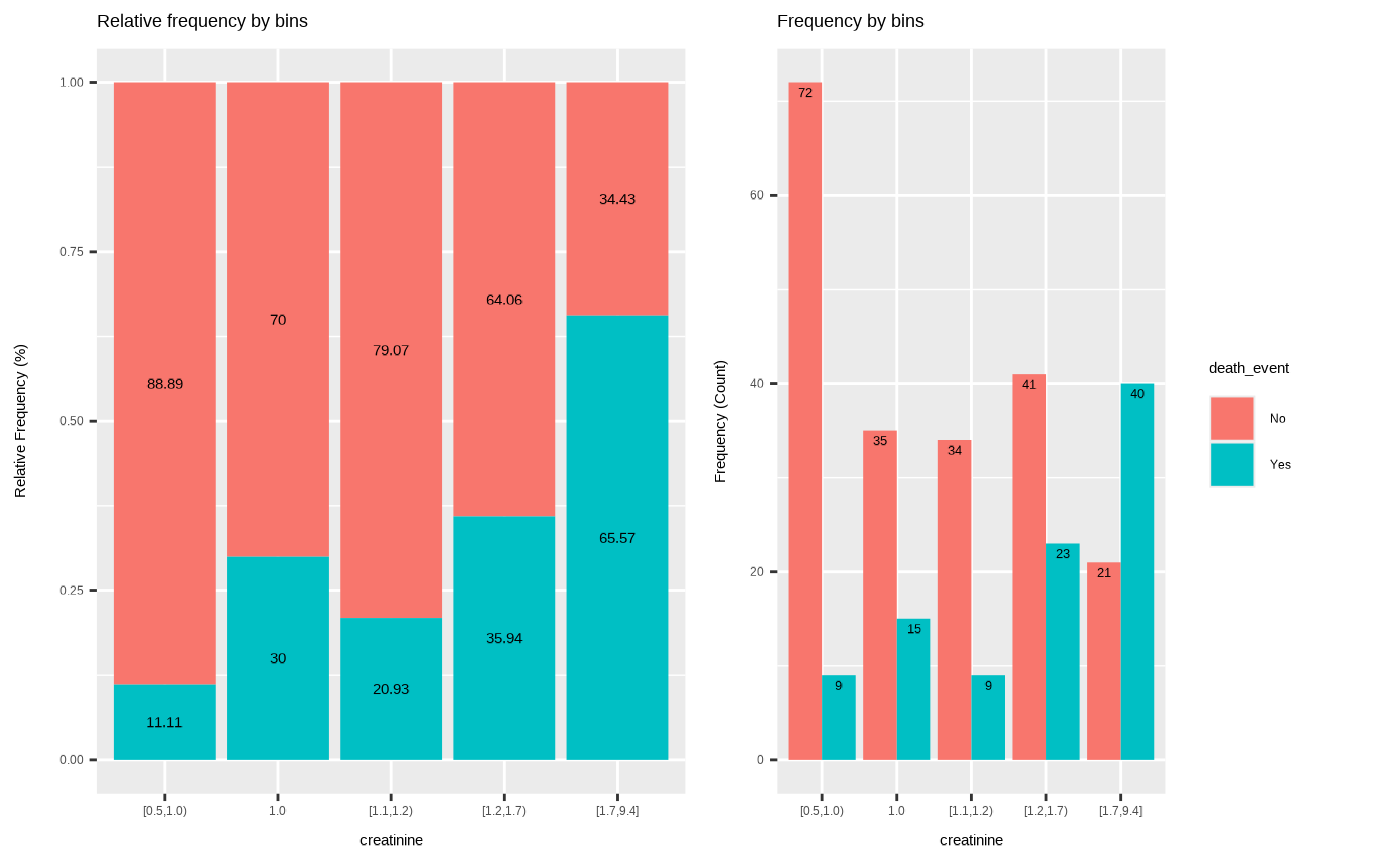The binning_rgr() finding intervals for numerical variable using recursive information gain ratio maximization.

binning_rgr(.data, y, x, min_perc_bins = 0.1, max_n_bins = 5, ordered = TRUE)

## Arguments

.data a data frame. character. name of binary response variable. The variable must character of factor. character. name of continuous characteristic variable. At least 5 different values. and Inf is not allowed. numeric. minimum percetange of rows for each split or segment (controls the sample size), 0.1 (or 10 percent) as default. integer. maximum number of bins or segments to split the input variable, 5 bins as default. logical. whether to build an ordered factor or not.

## Value

an object of "infogain_bins" class. Attributes of "infogain_bins" class is as follows.

• class : "infogain_bins".

• type : binning type, "infogain".

• breaks : numeric. the number of intervals into which x is to be cut.

• levels : character. levels of binned value.

• raw : numeric. raw data, x argument value.

• target : integer. binary response variable.

• x_var : character. name of x variable.

• y_var : character. name of y variable.

## Details

This function can be usefully used when developing a model that predicts y.

binning, binning_by, plot.infogain_bins.

## Examples

# \donttest{
library(dplyr)

# binning by recursive information gain ratio maximization using character
bin <- binning_rgr(heartfailure, "death_event", "creatinine")

# binning by recursive information gain ratio maximization using name
bin <- binning_rgr(heartfailure, death_event, creatinine)
bin#> binned type: infogain
#> number of bins: 5
#> x
#> [0.5,1.0)       1.0 [1.1,1.2) [1.2,1.7) [1.7,9.4]
#>        81        50        43        64        61
# summary optimal_bins class
summary(bin)#>      levels freq      rate
#> 1 [0.5,1.0)   81 0.2709030
#> 2       1.0   50 0.1672241
#> 3 [1.1,1.2)   43 0.1438127
#> 4 [1.2,1.7)   64 0.2140468
#> 5 [1.7,9.4]   61 0.2040134
# visualize all information for optimal_bins class
plot(bin)# visualize WoE information for optimal_bins class
plot(bin, type = "cross")# visualize all information without typographic
plot(bin, type = "cross", typographic = FALSE)# extract binned results
extract(bin) %>%
head(20)#>   [1.7,9.4] [1.1,1.2) [1.2,1.7) [1.7,9.4] [1.7,9.4] [1.7,9.4] [1.2,1.7)
#>   [1.1,1.2) [1.2,1.7) [1.7,9.4] [1.7,9.4] [0.5,1.0) [1.1,1.2) [1.1,1.2)
#>  1.0       [1.2,1.7) [0.5,1.0) [0.5,1.0) 1.0       [1.7,9.4]
#> Levels: [0.5,1.0) < 1.0 < [1.1,1.2) < [1.2,1.7) < [1.7,9.4]# }